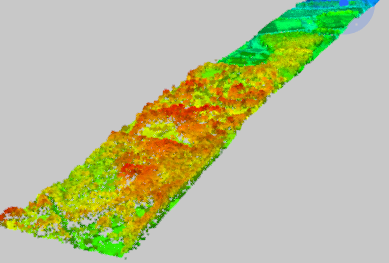Point Clouds (IFMEPointCloud)

A point cloud geometry is a (potentially large) collection of points. Point clouds are useful for storing large amounts of data, often gathered from LIDAR applications.Associated with each point are properties called components, which contain a value that describes the point. Components are similar to attributes, but at the point level instead of at the feature level. Note that all points in a point cloud have the same schema (that is, the same set of components), but each point may have a different value for each component.

All components have a name and type. Possible component types are Real64, Real32, UInt64, UInt32, UInt16, UInt8, Int64, Int32, Int16, Int8, and String. Numeric components additionally may have a scale and offset factor; in such cases, the applied value of the component is value x scale + offset. String components additionally may have an encoding.

While components may have any name, there are a few common components that exist in several formats. These components are described in the following table.

Note: This list is not exhaustive. A point cloud may have any number of components not listed here.

Component Name Description
x The x component of the geometry.
y The y component of the geometry.
z The z component of the geometry.
intensity The magnitude of the intensity of the pulse return.
color_red The red image channel value at the point.
color_green The green image channel value at the point.
color_blue The blue image channel value at the point.
classification The class of the point. Categorizes a point into fields, such as ground, building, water, and others. Values correspond to the ASPRS LAS specification.
return The pulse return number for a given output pulse.
number_of_returns The total number of detected returns from a single pulse.
gps_time The number of seconds since the beginning of the week.
gps_week The week number, counting from January 6th, 1980.
angle The angle of the pulse that the point was scanned at.
flight_line The flight line number the point was detected in.
flight_line_edge Whether this point lies on the edge of the scan, along the flight line.
scan_direction The direction in which a scanning mirror was directed when the point was detected.
point_source_id A value that indicates the source of the file, such as a file number.
posix_time Used to express the time, as the number of seconds elapsed since UTC January 1st, 1970.
user_data Data to be used at the user’s discretion.
normal_x The x component of the vertex normals.
normal_y The y component of the vertex normals.
normal_z The z component of the vertex normals.

Note that there are no required components. For example, point clouds typically have all of the x/y/z components, but a point cloud may not have z (making it 2D), or may not have any of x/y/z (making it non-spatial).

Point clouds may also store a 4x4 transformation matrix, which is applied to the x/y/z components.

Point Cloud Attributes

• fme_geometry = fme_polygon
• fme_type = fme_point_cloud